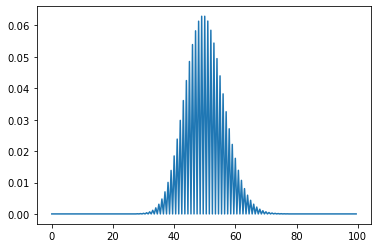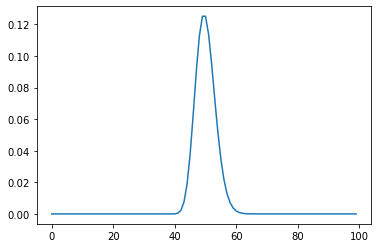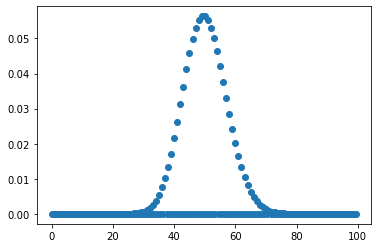# How to Create a Poisson Probability Mass Function Plot in Python?

• Last Updated : 13 Jan, 2022

In this article, we will see how we can create a Poisson probability mass function plot in Python. In probability theory and statistics, the Poisson distribution is a discrete probability distribution that expresses the probability of a given number of events occurring in a fixed interval of time or space if these events occur with a known constant mean rate and independently of the time since the last event. The Poisson distribution can also be used for the number of events in other specified intervals such as distance, area or volume.

In order to plot the Poisson distribution, we will use scipy module. SciPy is a free and open-source Python library used for scientific computing and technical computing. SciPy contains modules for optimization, linear algebra, integration, interpolation, special functions, FFT, signal and image processing, ODE solvers and other tasks common in science and engineering.

In order to get the poisson probability mass function plot in python we use scipy’s poisson.pmf method.
Syntax : poisson.pmf(k, mu, loc)
Argument : It takes numpy array, shape parameter and location as argument
Return : It returns numpy array

Example 1:

## Python3

 `# importing poisson from scipy``from` `scipy.stats ``import` `poisson` `# importing numpy as np``import` `numpy as np` `# importing matplotlib as plt``import` `matplotlib.pyplot as plt`  `# creating a numpy array for x-axis``x ``=` `np.arange(``0``, ``100``, ``0.5``)` `# poisson distribution data for y-axis``y ``=` `poisson.pmf(x, mu``=``40``, loc``=``10``)`  `# plotting the graph``plt.plot(x, y)` `# showing the graph``plt.show()`

Output :Example 2: Using step size of data as 1

## Python3

 `# importing poisson from scipy``from` `scipy.stats ``import` `poisson` `# importing numpy as np``import` `numpy as np` `# importing matplotlib as plt``import` `matplotlib.pyplot as plt`  `# creating a numpy array for x-axis``# using step size as 1``x ``=` `np.arange(``0``, ``100``, ``1``)` `# poisson distribution data for y-axis``y ``=` `poisson.pmf(x, mu``=``10``, loc``=``40``)`  `# plotting the graph``plt.plot(x, y)` `# showing the graph``plt.show()`

Output:Example 3: Plotting scatterplot for better viewing of data points

## Python3

 `# importing poisson from scipy``from` `scipy.stats ``import` `poisson` `# importing numpy as np``import` `numpy as np` `# importing matplotlib as plt``import` `matplotlib.pyplot as plt`  `# creating a numpy array for x-axis``x ``=` `np.arange(``0``, ``100``, ``0.5``)` `# poisson distribution data for y-axis``y ``=` `poisson.pmf(x, mu``=``50``, loc``=``0``)`  `# plotting thescatter plot graph``plt.scatter(x, y)` `# showing the graph``plt.show()`

Output:My Personal Notes arrow_drop_up